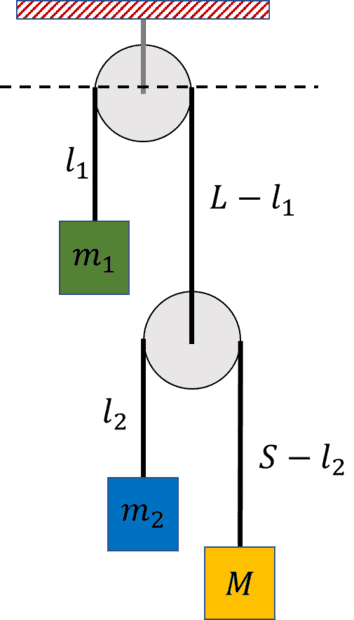# System of Masses - Atwood Machine

Gold MemberWould anyone verify whether or not I've formulated the proper Lagrangian here for the system above (the pulleys are massless, inextensible ropes of length ##L## and ##S##):

$$\mathcal{L} = T - U$$

$$\mathcal{L} = \frac{1}{2} m_1 { \dot l_1 }^2+ \frac{1}{2} m_2 \left( { \dot l_2}- {\dot l_1 } \right)^2 + \frac{1}{2} M \left( {\dot l_1}+ {\dot l_2} \right) ^2 + m_1 g l_1 + m_2 g ( L - l_1 + l_2) + M g ( L - l_1 + S - l_2 )$$

If I find out this step looks ok, I'll post the remainder. I'm getting some unexpected result at the end for ## \ddot{l_2}## when ##m_1 \to 0##, and I'm wondering if I'm making an algebra error in the middle, or I have made a mistake right from the get-go.

This is an extension of a recent HW problem that I proposed (and go figure... turns out I can't solve it myself). I figured I 'd start a new thread since this is a different method of analyzing it.

Thanks for any help.

Mentor
The Lagrangian looks good to me

Gold Member
The Lagrangian looks good to me
Thanks Dale! I'm going to piece this together so it's not too much to process. I'm a newbie to using this machinery.

Next step:

$$\frac{ \partial \mathcal{L} }{ \partial l_1 } = ( m_1 - m_2 -M ) g$$

$$\frac{ \partial \mathcal{L} }{ \partial l_2 } = ( m_2 -M ) g$$

$$\frac{ \partial \mathcal{L} }{ \partial \dot{l_1} } = m_1 \dot l_1 - m_2\left( \dot{l_2} - \dot{l_1} \right) + M \left( \dot{l_2} + \dot{l_1} \right)$$

$$\frac{ \partial \mathcal{L} }{ \partial \dot{l_2} } = m_2 \left( \dot{l_2} - \dot{l_1} \right) + M \left( \dot{l_1} + \dot{l_2} \right)$$

Gold Member
And:

$$\frac{d}{dt} \frac{ \partial \mathcal{L} }{ \partial \dot{l_1} } = \left ( m_1 + m_2+M \right) \ddot {l_1} + \left( M - m_2\right) \ddot {l_2}$$

$$\frac{d}{dt} \frac{ \partial \mathcal{L} }{ \partial \dot{l_2} } = \left( M - m_2 \right) \ddot{l_1} + \left( M+m_2 \right) \ddot{l_2}$$

Mentor
Yes, I get the same

Gold Member
This is not someone’s physics homework, why did I get a warning?

Mentor
You didn't get a point. I just moved it. Even though it isn't homework it is homework-like so it belongs here. I removed the points from the warning before moving it. It won't affect your membership at all

•erobz
Homework Helper
Gold Member
Are you going to solve the equations of motion or leave it at that?

Gold Member
Are you going to solve the equations of motion or leave it at that?
I’m planning on it, but since all that appears correct I’m thinking I have an algebra error to find…

•kuruman and Dale
Gold Member
I just had a lightbulb moment. It took many times putting into practice Einstein's definition of insanity... I didn't have an algebra mistake, but rather an interpretation error.

$$\frac{ \partial \mathcal{L} }{ \partial l_1 } = \frac{d}{dt} \frac{ \partial \mathcal{L} }{ \partial \dot{l_1} }$$

$$( m_1 - m_2 -M ) g = \left ( m_1 + m_2+M \right) \ddot {l_1} + \left( M - m_2\right) \ddot {l_2}$$

$$\ddot{l_1} = \frac{(m_1 -m_2 -M)g - (M-m_2) \ddot{l_2}}{m_1 + m_2 + M} \tag{1}$$

$$\frac{ \partial \mathcal{L} }{ \partial l_2 } = \frac{d}{dt} \frac{ \partial \mathcal{L} }{ \partial \dot{l_2} }$$

$$( m_2 -M ) g = \left( M - m_2 \right) \ddot{l_1} + \left( M+m_2 \right) \ddot{l_2} \tag{2}$$

Substitute ##(1) \to (2)##:

$$\ddot{l_2} = \frac{ (m_1 + m_2 + M)( m_2 - M ) - ( M - m_2)(m_1 -m_2 -M) }{ ( m_1 + m_2 + M )(M+m_2) -(M-m_2)^2 } g \tag{3}$$

I had expected the masses to be in freefall if ##m_1 \to 0##, I reached the conclusion that ##m_1 \to 0, \ddot{l_2} = g ##. That conclusion was reached in error.

I had found what I was looking for, but I just hadn't realized it ( because I was thinking in terms of what I had done in the HW problem trying to use Newtons Laws )

Instead, I see that as ##m_1 \to 0, \ddot{l_2} \to 0 g ## meaning the length of the rope is not changing w.r.t. the pulley its attached to. They are both accelerating at ## - \ddot{l_1} = g## which is found by substituting ##m_1 = 0, \ddot{l_2} = 0## into ##(1)##.

Thats very good news! (I think?)

P.S. I realize ##(3)## simplifies greatly...I was getting tired.

$$\ddot{l_2} = \frac{ 2m_1(m_2 - M) }{m_1M + m_1 m_2 + 2 M m_2}g \tag{3}$$

Last edited:
•Dale
Gold Member
What is not so comforting about arriving at this solution is my failure in applying Newtons Laws to solve this problem as I have tried in

Problem with 2 pulleys and 3 masses

Can anyone figure out what is wrong about that approach?

Last edited:
Mentor
post 10 looks good too

Gold Member
post 10 looks good too
I found an error in this one! That means the system is consistent

$$\ddot{l_2} = \frac{ 2m_1(m_2 - M) }{m_1M + m_1 m_2 + 2 M m_2}g \tag{3}$$
$$\ddot{l_2} = \frac{ 2m_1(m_2 - M) }{m_1M + m_1 m_2 + \boldsymbol{4} M m_2}g \tag{3}$$

Gold Member
Thanks for all the verification along the way @Dale.